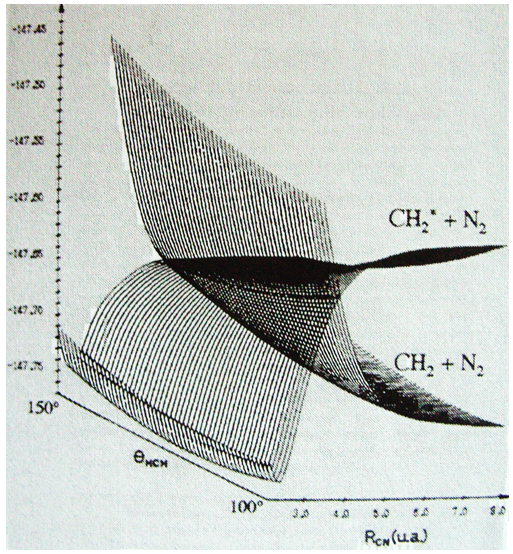# Chapter 10 : MPC – Molecules and Born-Oppenheimer

The Hamiltonian quickly becomes monstrously difficult when several atoms and electrons are considered. To illustrate this point, the equations of the Hamiltonians for H, H2+ and H2 are showed below: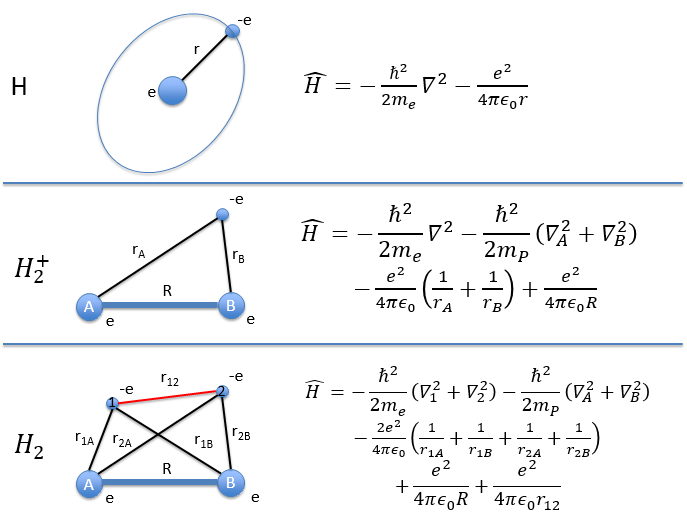For a molecule with M nuclei of atomic number Z1, Z2, Z3, …, ZM and n electrons, the global expression is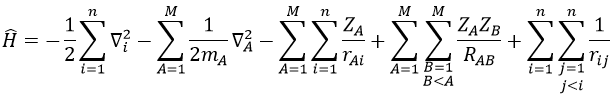The Hamiltonian can be decomposed into 5 terms, two from the kinetics of the electrons and nuclei and 3 for the interactions between the electron-electron, nucleus-nucleus and nucleus-proton.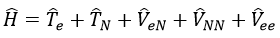As soon as there are several nuclei, there is no central field anymore and we can’t develop a spherical symmetry to build the CSCO. To simplify the problem, we use the Born-Oppenheimer approximation. The electrons have a mass that is way smaller than the one of nuclei (around 1800times smaller than the mass of a proton) and they move way faster. The approximation is to consider that the electrons are adapting themselves instantly to the movements of the nuclei and that we can consider the nuclei as immobile to determine the movements of the electrons.

As a result, we get one equation of Schrödinger for the electrons in the field of the fixed nuclei (TN=0).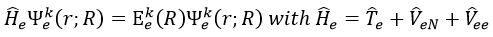The coordinates of the nuclei are a parameter and not a variable anymore in this equation. We can solve the equations for any position of the nuclei. Then we solve the Schrödinger’s equation for the nuclei.

We treat a single electronic state at a time.  It gives the surface of potential energy. We talk about a surface of potential energy but the dimension depends on the quantity of nuclei. The surface of potential energy depends on 3M-6 intern coordinates) (3M-5 for linear molecules) where M is the amount of nuclei. The -6 comes from the fact that the 3 degrees of translation and of rotation do not impact the potential energy. The global wave function of the molecule is the combination of the wave functions for the electrons and the nuclei. We can plot the potential of BO simply by the addition of the electronic energies as a function of the distance between the nuclei with the nucleic potential.

It forms the potential of Born-Oppenheimer that can show a minimum and the state is binding, or no minimum and the state is not binding: the most stable distance between the nuclei is infinite.

During a reaction, the distances between the nuclei vary to form a new liaison and we can plot isocurves of potential energy as a function of the internucleic distances but the approximation that the nuclei are immobile becomes bad as their movements are an important parameter of the problem. It is the case during the cleavage or the formation of a liaison.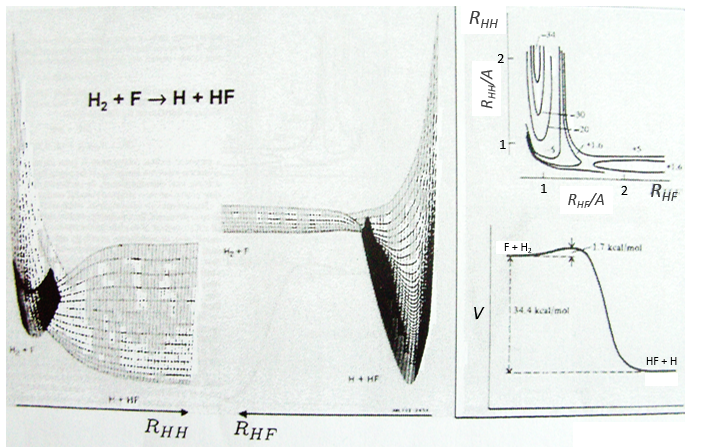Something that was neglected in the approximation of BO is that the different states can interact together and that the couplings between these states can be important. We isolated one state and neglected the couplings it can have with the other states. The exact solution takes them into account. The difference is especially important if two states are close in term of energy or if there is a crossing of the states of energy as a function of the distance between the nuclei (see below an example for the diazomethane, the parallel lines are for various angles of approach). In these cases the coupling terms are not negligible anymore and the approximation.